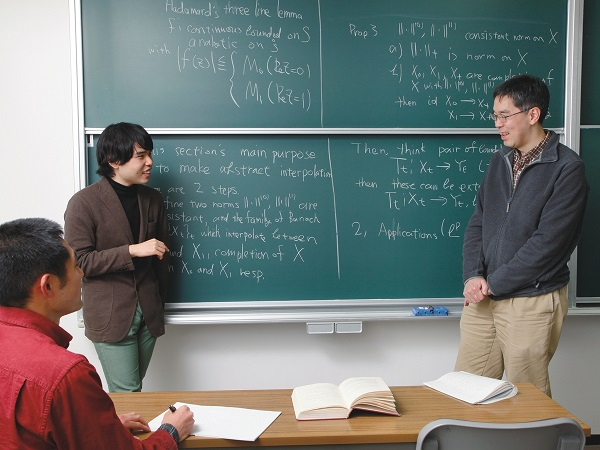Mathematics—an eye for reading natural phenomena - School of Science, the University of Tokyo
Oct 16, 2015

## 05. Department of Mathematics—Nakamura

Shu Nakamura
Professor
Mathematics ProgramProfessor Nakamura left a Ph.D. program at the University of Tokyo Graduate School of Science in 1985 to become an assistant professor at the University of Tokyo College of Arts and Sciences, a position he held until 1990. Then, he served as an associate professor until 1995. Following that he was a professor at the Nagoya University Graduate School of Mathematics until 1997, when he took up his current position at the University of Tokyo.

Mathematics is both a method and a language for understanding various natural phenomena. For example, Newton’s equations of motion give us a way to calculate what will happen when a point is traveling in space at a given speed. However, when we focus on outer space, the movement of the planets against the background of the stars is quite complex. It is difficult to use the simplified world described by the Newtonian equations to obtain a comprehensive understanding of such complex movement. However, no matter how complex these phenomena are, they can be understood by mathematics.

“The world described by mathematics is a simplified world, one that exists only in the minds of mathematicians,” Professor Shu Nakamura says. “But it’s also a ‘closed’ world that mathematicians can manipulate and study closely, giving them a better understanding of actual phenomena.”

Nakamura’s main interest is the Schrödinger equation, part of the mathematical structure of quantum mechanics. The Schrödinger equation is a universal basic equation corresponding to Newtonian equations in classical mechanics. It is said that almost all physical phenomena and physical properties can ultimately be described by this equation.

Currently, research is focusing on analysis of the Schrödinger equation on Riemannian manifolds by using scattering theory and microlocal analysis, and on analysis of Schrödinger operators related to condensed matter physics. In particular, scattering operators that can be investigated by functional analysis and microlocal analysis are extremely important for obtaining a mathematical understanding of problems related to nuclear theory.

When one thinks of mathematics, the drama of solving unanswered problems is one thing that comes to mind. A good example is Fermat’s last theorem, an extremely difficult problem from the seventeenth century. Mathematician Andrew Wiles captured the world’s attention when he finally provided a complete proof of it in 1995.

A similar outstanding problem regarding the Schrödinger equation is whether a continuous spectrum for the Anderson model exists. If this is found it will provide a fundamental explanation for why a finite current flows in matter, but it is an extremely difficult problem that has not been solved in the decades since it was proposed.

“Solving big problems is one of the great things that a mathematician can do,” Nakamura says, “but in a sense solving small problems is the same, in that both aid the understanding of some phenomenon. Answers to problems are accompanied by better understanding of various other peripheral issues. The important thing is that it expands the borders of our understanding. The path to solving a big problem is paved with the repeated solving of smaller ones.” To a mathematician, there is drama in the understanding that comes from solving both large and small problems.

Mathematical research carries an image of isolation, but Nakamura says, “I don’t think many mathematicians would last long without someone around who considers what they do interesting. Research relies on community.” There are some goals that are too large for any one person to handle. Seminars that proceed through student discussions do not feel dark and isolated. “Good teachers help us notice areas that we don’t really understand,” says Tatsuhiko Miura, a fourth-year undergraduate student. Seminars take place through discussions after reading about mathematics in textbooks, and discussions cover far more material than is presented in the book. Filling in the gaps between what’s written in the text and a full understanding is the job of the learner. Doing so provides good practice that develops a base for one’s own research on mathematics.

We have extensive international contacts with co-researchers in France, the UK, Spain, and other countries around the world. Mathematics is not just a language for understanding the world; it’s also one for bringing the world together.

Student Evaluations

“I sometimes make huge mistakes, but this professor never gives up on me. I’m very thankful for that.” (Keita Mikami, B4)

“He gives plenty of time for discussion, which makes things clearer and easier to understand.” (Tatsuhiko Miura, B4)The Schrödinger equation was proposed in 1926 by Austrian physicist Erwin Schrödinger as a fundamental equation for wave mechanics in quantum mechanics. It is an equation that describes the time evolution of quantum states.

― Office of Communication ―